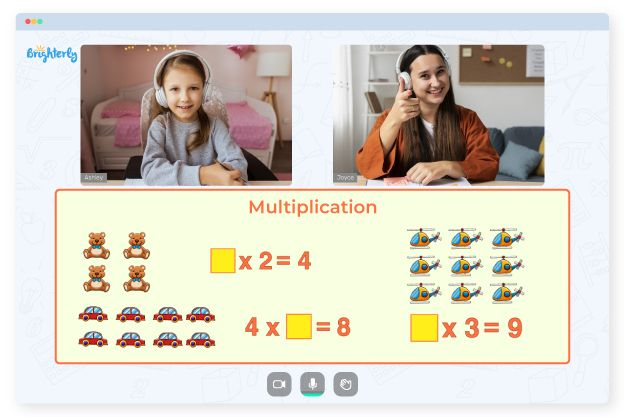# Multiplication Facts Worksheets

Multiplication is an essential aspect of math. It is the foundation for solving numerous complex world-problem-solving equations we encounter today. Kids must learn the basics of multiplication when they are younger; they should understand word problems, multiple-choice questions, and other types of tasks within the multiplication universe.

Multiplication fact worksheets visually represent all the multiplication problems that kids will encounter in their grade, and the sheets have answer keys to help kids get through each question and solution. Parents, teachers, tutors, and kids use multiplication fact worksheets as a guide when navigating the world of multiplication.

## Benefits of multiplication fact worksheets

Math facts worksheets multiplication have many benefits for kids, parents, and teachers, and sometimes tutors also have to work with these tools. Here are some of the benefits of multiplication fact practice worksheets:

### They help kids remember the facts

Multiplication facts work more with remembrance than they do with equations. Kids do not necessarily need to solve the facts, but they must remember them to solve other multiplication problems. With the multiplication facts worksheet, kids can remember the facts they have seen and practiced over time. The more they practice with these worksheets, the more their brains work hard to save the essential things they repeat: multiplication facts. With math facts worksheets multiplication becomes easier for kids to understand.

### They allow parents understand how to help their kids

Parents often find themselves complaining about the way math processes go these days. With math facts worksheets multiplication processes get easier for parents trying to teach their kids. Parents often need extra aid when helping kids with homework and study time. The multiplication facts practice worksheets don’t just teach kids what to do; they teach parents how to deal with math problems too.

### They help teachers set homework

Teachers often struggle with effective homework for kids because they have to ensure that the assignment aligns with what they have taught in class and still challenges students. For example, math facts multiplication worksheets may have over 30 practice questions that touch on all the aspects of multiplication facts.

Math for Kids

Is Your Child Struggling With Math?
1:1 Online Math TutoringSo, teachers do not have to worry that the homework is not all-encompassing. All they have to do is give out the same worksheets that touch on the multiplication issues they are dealing with for that week. More importantly, they can go through the answered worksheets to see how each kid solved their problems and what concepts they are struggling with.

### They help tutors understand students’ level better

Before a tutor takes up the task of teaching a kid math, they have to first understand what issues each child may be having with different math concepts so that they can turn those weaknesses into strengths. For example, using the multiplication facts worksheet, a tutor can ascertain where a child is struggling by how they answer the questions on the sheet and what answers they got right. A tutor’s job is complete when they successfully address the learner’s weaknesses.

Numerous multiplication fact families worksheets are available on the internet, specifically on math websites. So, instead of going through the stress of creating one from scratch, you can download any multiplication facts worksheet you like. It would be better for you to pick the fun ones with colors and objects that catch your kids’ attention and keep them interested in the idea.### Multiplication Facts Worksheets PDF

Multiplication Fact Practice Worksheets### Multiplication Facts Worksheets PDF

Multiplication Fact Worksheets### Multiplication Facts Worksheets PDF

Multiplication Facts Worksheets PDF### Multiplication Facts Worksheets PDF

Multiplication Math Facts Worksheets

Need help with Multiplication?• Is your child having trouble mastering the fundamentals of multiplication?
• Try studying with an online tutor.

Does your child need additional help in mastering multiplication? Start learning with an online tutor.